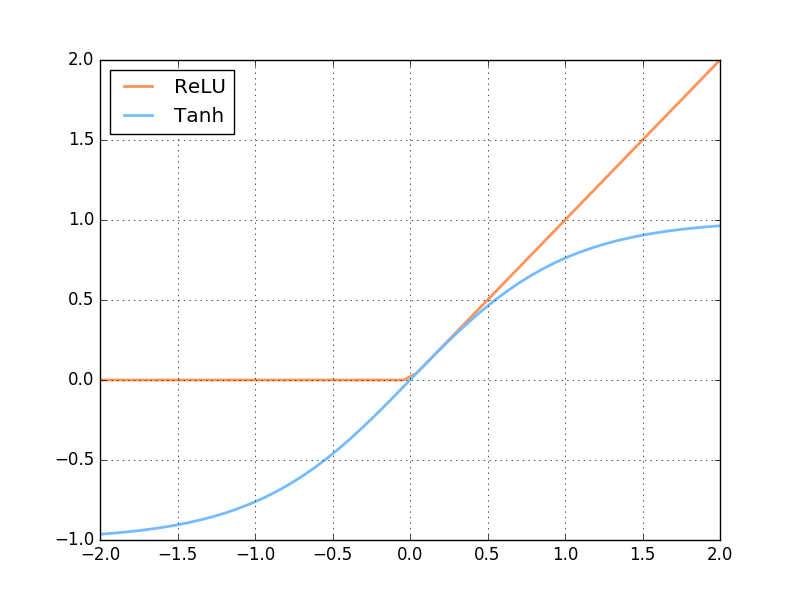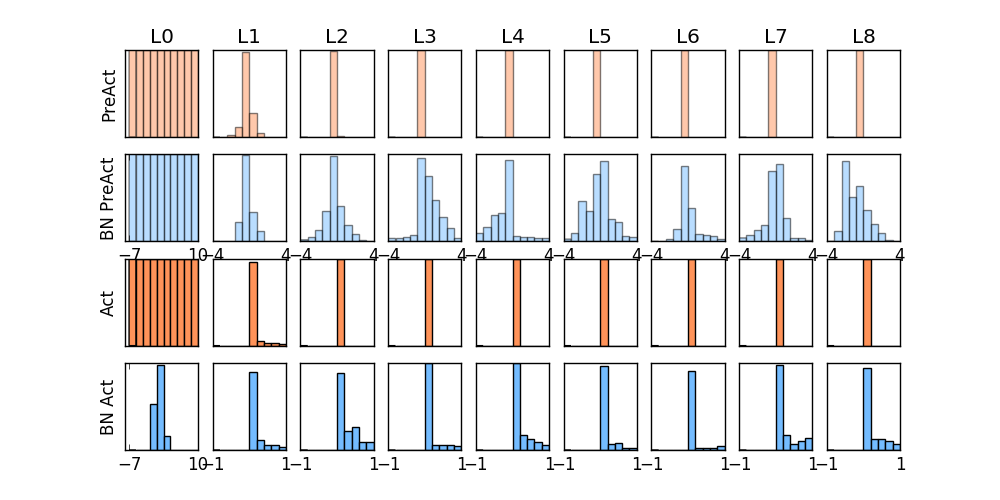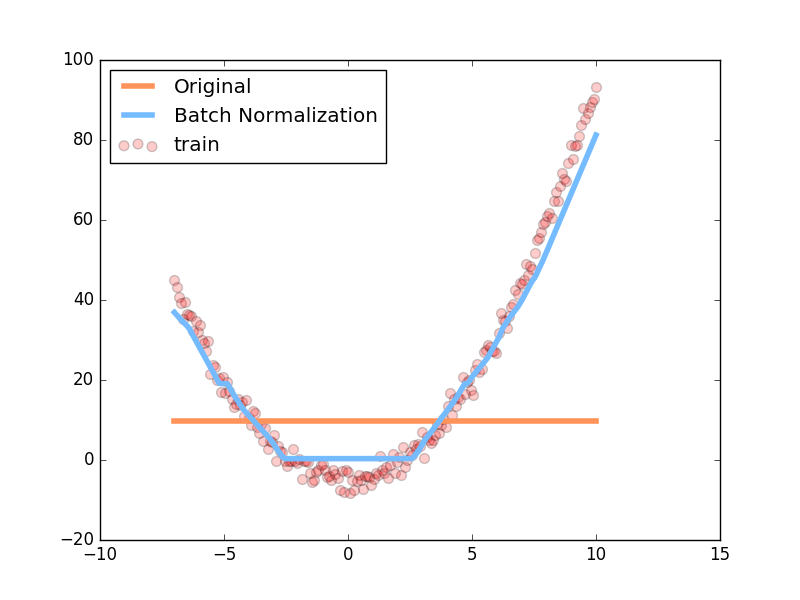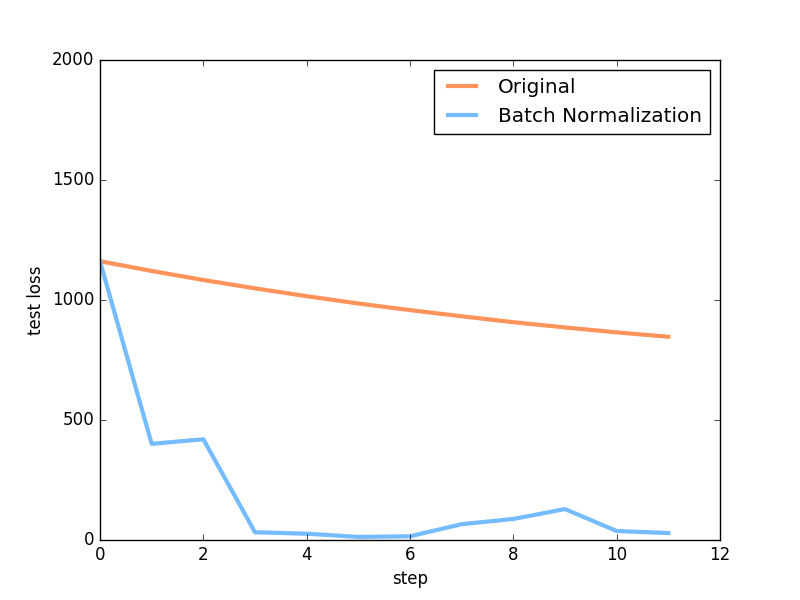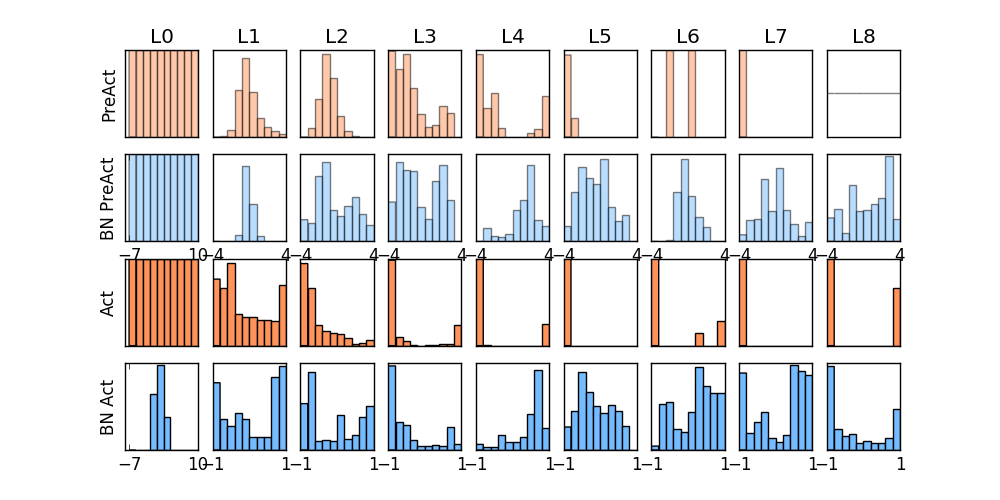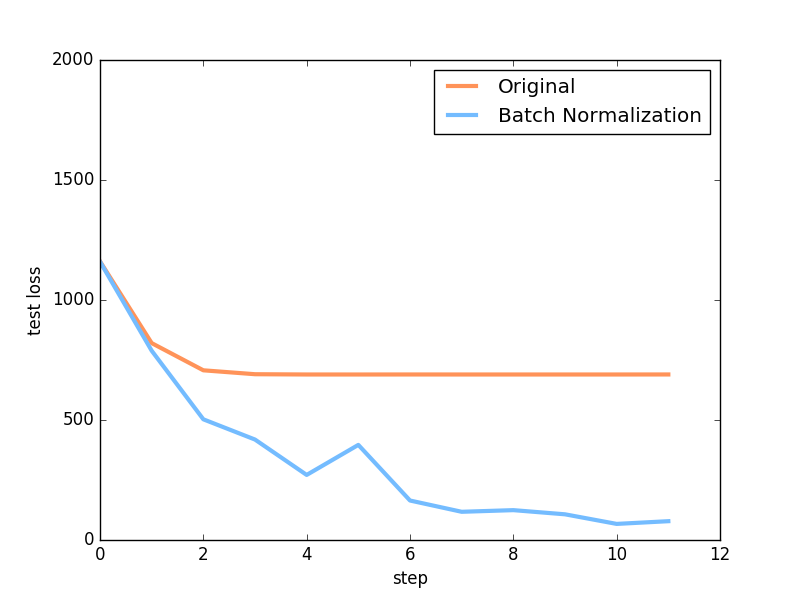#### 学习资料:

Batch Normalization 批标准化：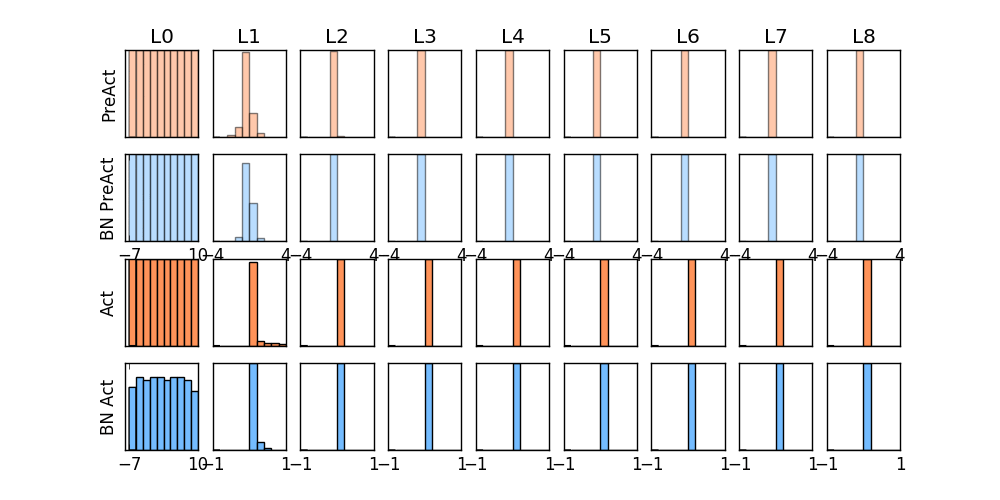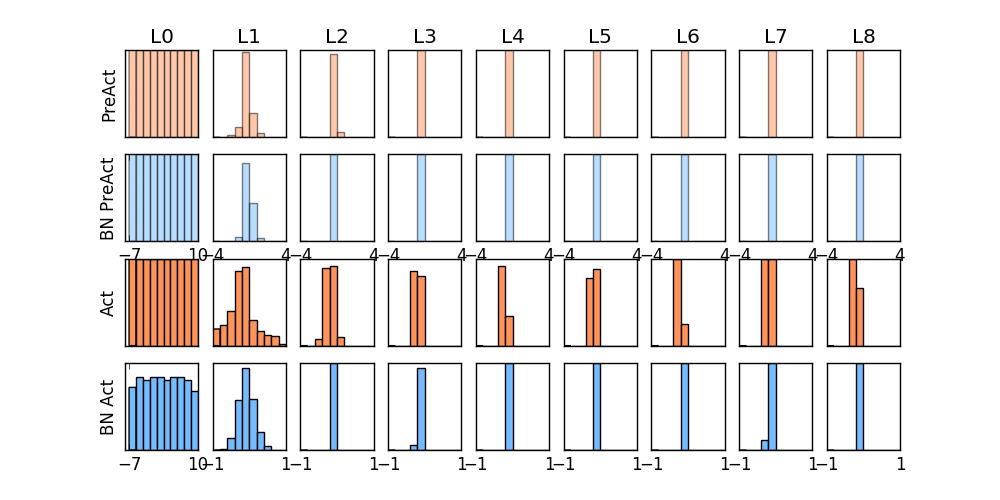##### 做点数据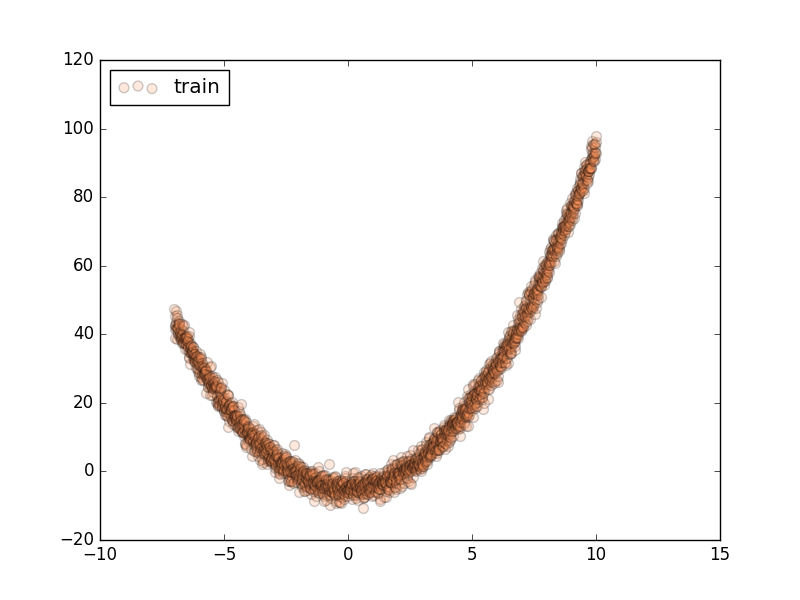``````import torch
from torch import nn
from torch.nn import init
import torch.utils.data as Data
import torch.nn.functional as F
import matplotlib.pyplot as plt
import numpy as np

# 超参数
N_SAMPLES = 2000
BATCH_SIZE = 64
EPOCH = 12
LR = 0.03
N_HIDDEN = 8
ACTIVATION = F.tanh     # 你可以换 relu 试试
B_INIT = -0.2   # 模拟不好的 参数初始化

# training data
x = np.linspace(-7, 10, N_SAMPLES)[:, np.newaxis]
noise = np.random.normal(0, 2, x.shape)
y = np.square(x) - 5 + noise

# test data
test_x = np.linspace(-7, 10, 200)[:, np.newaxis]
noise = np.random.normal(0, 2, test_x.shape)
test_y = np.square(test_x) - 5 + noise

train_x, train_y = torch.from_numpy(x).float(), torch.from_numpy(y).float()
test_x = Variable(torch.from_numpy(test_x).float(), volatile=True)  # volatile=True 不进行梯度计算
test_y = Variable(torch.from_numpy(test_y).float(), volatile=True)

train_dataset = Data.TensorDataset(data_tensor=train_x, target_tensor=train_y)

# show data
plt.scatter(train_x.numpy(), train_y.numpy(), c='#FF9359', s=50, alpha=0.2, label='train')
plt.legend(loc='upper left')
plt.show()
``````
##### 搭建神经网络

``````class Net(nn.Module):
def __init__(self, batch_normalization=False):
super(Net, self).__init__()
self.do_bn = batch_normalization
self.fcs = []   # 太多层了, 我们用 for loop 建立
self.bns = []
self.bn_input = nn.BatchNorm1d(1, momentum=0.5)   # 给 input 的 BN

for i in range(N_HIDDEN):               # 建层
input_size = 1 if i == 0 else 10
fc = nn.Linear(input_size, 10)
setattr(self, 'fc%i' % i, fc)       # 注意! pytorch 一定要你将层信息变成 class 的属性! 我在这里花了2天时间发现了这个 bug
self._set_init(fc)                  # 参数初始化
self.fcs.append(fc)
if self.do_bn:
bn = nn.BatchNorm1d(10, momentum=0.5)
setattr(self, 'bn%i' % i, bn)   # 注意! pytorch 一定要你将层信息变成 class 的属性! 我在这里花了2天时间发现了这个 bug
self.bns.append(bn)

self.predict = nn.Linear(10, 1)         # output layer
self._set_init(self.predict)            # 参数初始化

def _set_init(self, layer):     # 参数初始化
init.normal(layer.weight, mean=0., std=.1)
init.constant(layer.bias, B_INIT)

def forward(self, x):
pre_activation = [x]
if self.do_bn: x = self.bn_input(x)    # 判断是否要加 BN
layer_input = [x]
for i in range(N_HIDDEN):
x = self.fcs[i](x)
pre_activation.append(x)    # 为之后出图
if self.do_bn: x = self.bns[i](x)  # 判断是否要加 BN
x = ACTIVATION(x)
layer_input.append(x)       # 为之后出图
out = self.predict(x)
return out, layer_input, pre_activation

# 建立两个 net, 一个有 BN, 一个没有
nets = [Net(batch_normalization=False), Net(batch_normalization=True)]
``````
##### 训练

``````opts = [torch.optim.Adam(net.parameters(), lr=LR) for net in nets]

loss_func = torch.nn.MSELoss()

losses = [[], []]  # 每个网络一个 list 来记录误差
for epoch in range(EPOCH):
print('Epoch: ', epoch)
for step, (b_x, b_y) in enumerate(train_loader):
b_x, b_y = Variable(b_x), Variable(b_y)
for net, opt in zip(nets, opts):     # 训练两个网络
pred, _, _ = net(b_x)
loss = loss_func(pred, b_y)
loss.backward()
opt.step()    # 这也会训练 BN 里面的参数
``````
##### 画图``````f, axs = plt.subplots(4, N_HIDDEN+1, figsize=(10, 5))

def plot_histogram(l_in, l_in_bn, pre_ac, pre_ac_bn):
...

for epoch in range(EPOCH):
layer_inputs, pre_acts = [], []
for net, l in zip(nets, losses):
# 一定要把 net 的设置成 eval 模式, eval下的 BN 参数会被固定
net.eval()
pred, layer_input, pre_act = net(test_x)
l.append(loss_func(pred, test_y).data)
layer_inputs.append(layer_input)
pre_acts.append(pre_act)
# 收集好信息后将 net 设置成 train 模式, 继续训练
net.train()
plot_histogram(*layer_inputs, *pre_acts)     # plot histogram

# 后面接着之前 for loop 中的代码来
for step, (b_x, b_y) in enumerate(train_loader):
...
``````

##### 对比结果